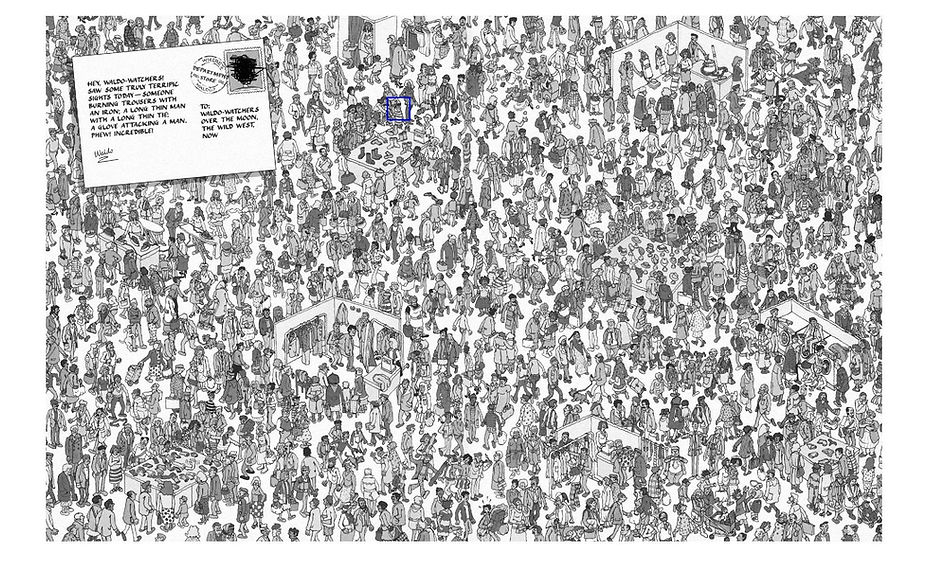## Step #1

Exploration #1

Cross Correlation w/Waldo

Using a cross correlation of Waldo's face with a "Where's Waldo" image in order to locate Waldo.

Exploration #2

Feature Matching

Using feature matching functions built into MATLAB in order to match an image of Waldo to his location in a "Where's Waldo" image.

Exploration #3

SVD Compression

Attempt to address the problems from explorations 1 and 2 using SVD compression.

## Explorations Overview

In this exploration, we took a cropped greyscale image of Waldo and cross correlated it with a greyscale "Where's Waldo" image. After which the location of the peak value is determined and indicated at the output.

## Exploration #1: Cross Correlation w/Waldo

clear all

clc

figure(1)

GeneralView=rgb2gray(example2);

Waldo=rgb2gray(CroppedWaldo2);

%Correlation

cc=normxcorr2(Waldo, GeneralView);

mesh(cc)

figure(2)

imshow(GeneralView)

[maxval]=max(max(abs(cc)))

[xpeak,ypeak]=find(cc==maxval)

hold on;

rectangle('Position',[(xpeak-50) (ypeak-50) 75 75],'LineWidth',2,'EdgeColor','b');

Figure 1 shows a cropped image of Waldo along with the image it was taken from. In figure 2 it can be seen that the algorithm correctly identifies the location of Waldo from the original image.Figure 1: Cross Correlation Image (left) taken from image we are searching (right)

Figure 2: Algorithm correctly identifying WaldoIn this exploration, we used the built in detectHarrisfeatures function in MATLAB which matches corners in the images in order to match a cropped image of Waldo taken from a "Where's Waldo" image. The result was similar to that of exploration #1, in which unless the cropped image was taken directly from the image we are searching in, the output would not be correct.

## Exploration #2: Feature MatchingFigure 4 shows a positively identified image of Waldo using the detectHarrisfeatures function. Figure 5 shows the problem whereusing a different image of Waldo's face than the one in the image we are searching yields several potential locations of Waldo.Figure 4: Waldo positively identified using detectHarrisfeatures and image of Waldo's face from the image we are searching.

Figure 5: Many potential Waldo locations identified using detectHarrisfeatures and a different image of Waldo's face than the one in the image we are searching.

This exploration was an attempt to address the problem of not being able to match an image of Waldo to the Waldo in a picture unless the two are exactly the same. In particular, one of the problems had to do with the different sized "Where's Waldo" images. This SVD compression function would lower the rank of the "Where's Waldo" image so that it could then be cross correlated.

## Exploration #3: SVD Compression

function [ Return ] = DenoisingSVD(Matriz,rank)

A=zeros(size(Matriz,1),size(Matriz,2));

%Matriz=rgb2gray(Matriz);

for i=1:size(Matriz,1)

for j=1:size(Matriz,2)

A(i,j)=(double(Matriz(i,j)))/255;

end

end

[U,S,V]=svd(A);

WaldoKrank=U(:,1:rank)*S(1:rank,1:rank)*V(:,1:rank)';

Return=uint8(255*WaldoKrank);

end